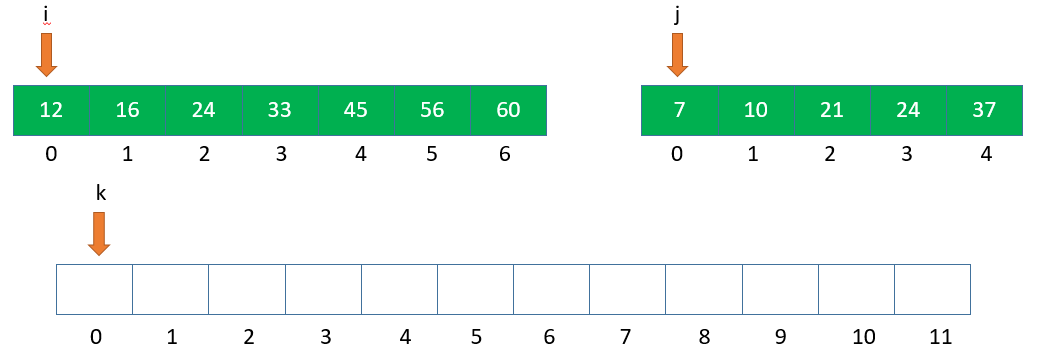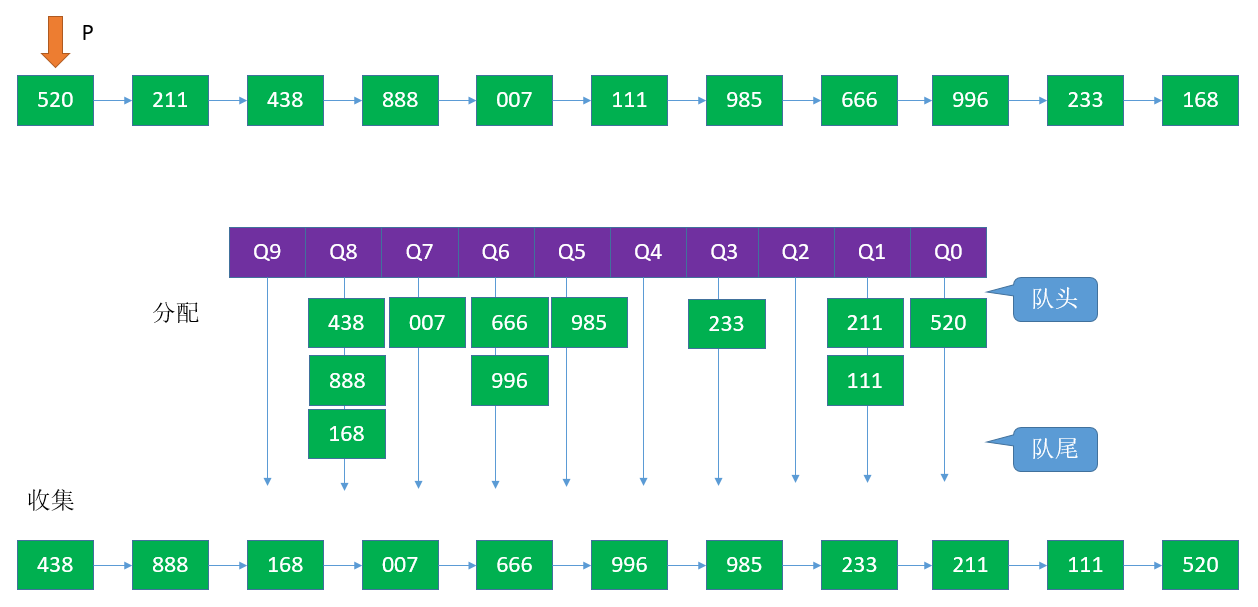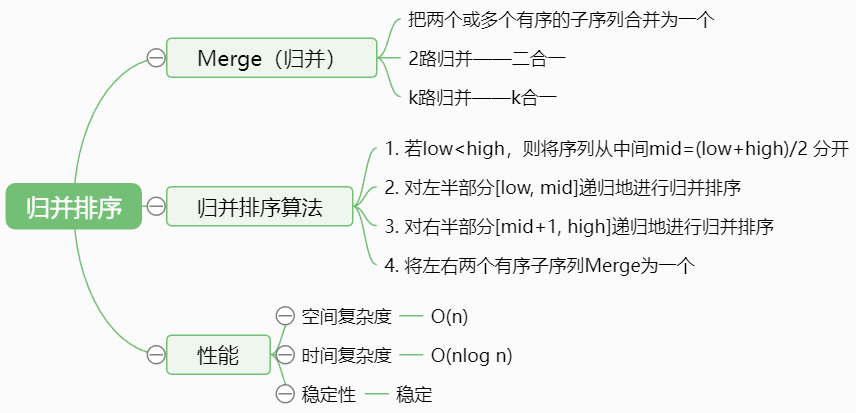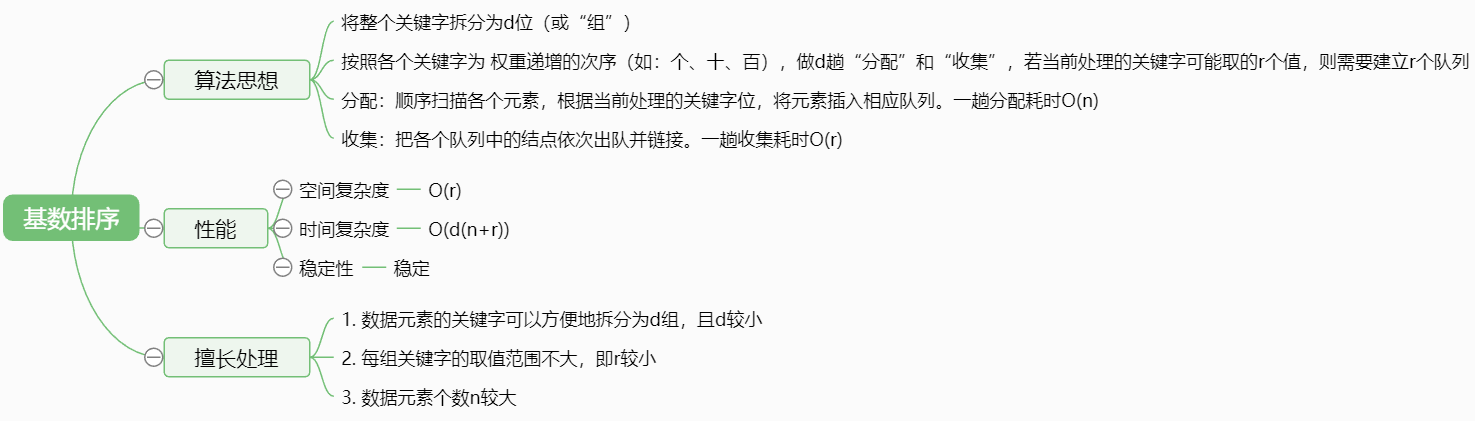V1

2023/04/05阅读：39主题：萌绿

# 【数据结构】归并排序、基数排序

### 1. 归并排序

#### 1. 归并排序的定义“2路”归并—每选出一个小元素需要对比关键字1次。

m路归并，每选出一个元素需要对比关键字m-1次

#### 3. 算法实现

``int *B=(int *)malloc(n*sizeof(int));  // 辅助数组B// A[low,...,mid]和A[min+1,...,high]各自有序，将两部分归并void Merge(int A[],int low,int mid,int high){    int i,j,k;    for(k=low;k<=high;k++){        B[k]=A[k];  // 将A中所有元素复制到B中    }    for(i=low,j=mid+1,k=i;i<=mid && j<=high;k++){ // 归并        if(B[i]<=B[j]){            A[k]=B[i++];  // 将较小值复制到A中。两个元素相等时，优先使用靠前的那个（稳定性）        }else{            A[k]=B[j++];        }    }    while(i<=mid){        A[k++]=B[i++];    }    while(j<=high){        A[k++]=B[j++];    }}void MergeSort(int A[],int low,int high){    if(low<high){        int mid=(low+high)/2;  // 从中间划分        MergeSort(A,low,mid); // 对左半部分归并排序        MergeSort(A,mid+1,high);  // 对右半部分归并排序        Merge(A,low,mid,high);  // 归并    }}``

#### 4. 算法效率分析

2路归并“归并树”——形态上就是一棵倒立的二叉树。

### 1. 过程模拟说明：首先观察，个位可以取到0-9的9个数。指针P从前往后扫描，第一趟将个位的数字分配到对应的队列中，然后收集。第二趟按照十位采用相同的方法分配和收集，第三趟按照百位采用相同的方法分配和收集，最终得到了一个降序排列的序列。

#### 2. 基数排序

1. 初始化:设置r 个空队列，Qr-1, Qr-2,...,Q0
2. 按照各个关键字位权重递增的次序(个、十、百)，对d个关键字位分别做“分配”和“收集”。
3. 分配:顺序扫描各个元素，若当前处理的关键字位=x，则将元素插入Qx队尾。
4. 收集:把Qr-1, Qr-2,...,Q0各个队列中的结点依次出队并链接。

#### 4. 基数排序的应用

1. 数据元素的关键字可以方便地拆分为d组，且d较小。
2. 每组关键字的取值范围不大，即r较小。
3. 数据元素个数n较大。

### 3. 小结V1